## Tuesday, March 24, 2015

### Splitting Audio Word Files into Syllables and Plotting Syllabic FFT Frequencies in MATLAB

Problem

In a previous post, we discussed how to read in an audio file recording a single word and plot it in MATLAB. In this post, we will discuss how split an audio file with a single word into syllables and plot syllabic frequencies obtained from the FFT analysis in MATLAB. We will again work with this audio file (calcium.wav) that has a recording of the word calcium. We will assume that the file is saved in C:\Users\Vladimir\audio_files

Elimination of Silence at the Beginning and End

We start by adding a path to the path array and reading in the audio file with MATLAB's audioread.

[x, fs] = audioread('calcium.wav'); %% x is the signal vector, fs is the frequency

Since this is a stereo file, we extract the signal vectors from each channel. They are identical in this case, so we will work with the vector from the first channel.

x_01 = x(:,1); %% get the signal vector of the 1st channel
x_02 = x(:,2); %% get the signal vector of the 2nd channel

The frequency range factor variable is defined to make our frequency ranges below more plottable.

frf = 1000; %% frequency reduction factor

If we look at the plot of the word calcium in this post, we will notice that there are silences at the beginning and end of the audio channel. We can estimate the beginning and end of the actual word and eliminate silence on both ends. The values 5.51e4 and 8.89e4 are our estimates of the beginning and end of the actual word.

figure(1);

word_01 = x_01(5.51e4:8.89e4);

We define the time line of the actual word. The time line goes from 0 upto (length(word_01)-1)/fs in increments of 1/fs, which is a common formula used in many audio processing applications. Once we have a time line, we can plot the amplitude of the recorded word against that time line.

t_word_01 = 0:1/fs:(length(word_01)-1)/fs;
plot(t_word_01, word_01);
xlabel('Time (seconds)');
ylabel('Amplitude');
title('{\bf Plot of the Word Calcium}')

Figure 1 shows the plot.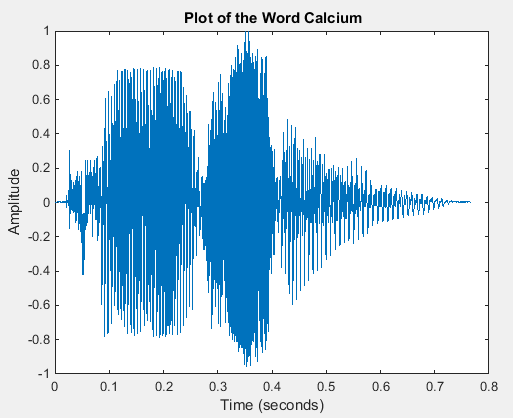Figure 1. Time vs. Amplitude Plot of the Word Calcium

Extracting and Plotting Syllables

The syllable audio segments can be extracted by looking at the plot in Figure 1. One can think of fs as speed. The whole audio vector becomes a distance segment. To get a specific distance point in the segment, we multiply time by speed. Thus, the first syllable occurs roughly between 0.025*fs and 0.26*fs. Once we have the syllable we can extract it, define its time line, and plot it.

syl_01_word_01 = word_01(0.025*fs:0.26*fs);
t_syl_01_word_01 = (0:1/fs:(length(syl_01_word_01)-1)/fs);
figure(2);
plot(t_syl_01_word_01, syl_01_word_01);
xlim([0 t_syl_01_word_01(end)]);
xlabel('Time (seconds)');
ylabel('Amplitude');
title('{\bf Plot of the Syllable CAL}');

We can verify the accuracy of our syllabic boundaries by playing the extracted segment.

soundsc(syl_01_word_01, fs);

Figure 2 shows the time vs. amplitude plot of the first syllable CAL (KAL).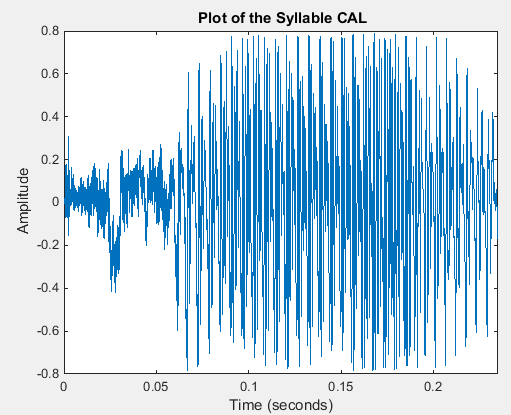Figure 2. Time vs. Amplitude Plot of the 1st Syllable CAL

The exact same techniques are used to extract the second (SI) and third syllabi (UM) and to plot them.

syl_02_word_01 = word_01(0.27*fs:0.51*fs);
t_syl_02_word_01 = (0:1/fs:(length(syl_02_word_01)-1)/fs);
figure(3);
plot(t_syl_02_word_01, syl_02_word_01);
xlim([0 t_syl_02_word_01(end)]);
xlabel('Time (seconds)');
ylabel('Amplitude');
title('{\bf Plot of the Syllable SI}');

syl_03_word_01 = word_01(0.51*fs:0.71*fs);
t_syl_03_word_01 = (0:1/fs:(length(syl_03_word_01)-1)/fs);
figure(4);
plot(t_syl_03_word_01, syl_03_word_01);
xlim([0 t_syl_03_word_01(end)]);
xlabel('Time (seconds)');
ylabel('Amplitude');
title('{\bf Plot of the Syllable UM}');

Figures 3 and 4 show the plots obtained by running the above MATLAB code.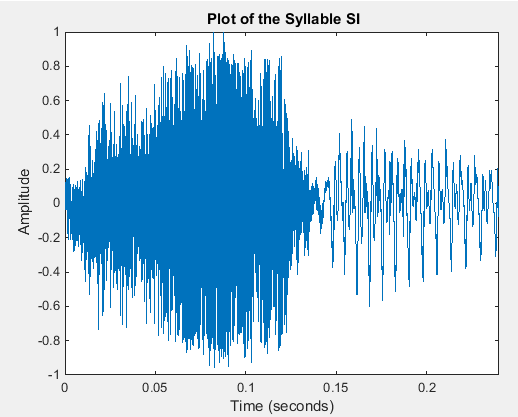Figure 3. Time vs. Amplitude Plot of the Second Syllable SI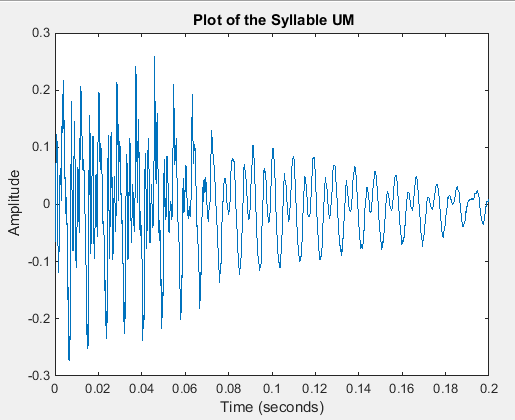Figure 4. Time vs. Amplitude Plot of the Third Syllable UM

After the syllables are extracted, we extract and plot individual consonants, i.e., K, S, and M, from the first, second, and third syllabi, respectively.

k_word_01 = word_01(0.019*fs:0.09*fs);
t_k_word_01 = (0:1/fs:(length(k_word_01)-1)/fs);
figure(5);
plot(t_k_word_01, k_word_01);
xlim([0 t_k_word_01(end)]);
xlabel('Time (seconds)');
ylabel('Amplitude');
title('{\bf Plot of the Consonant K}');
%soundsc(k_word_01, fs);

s_word_01 = word_01(0.27*fs:0.40*fs);
t_s_word_01 = (0:1/fs:(length(s_word_01)-1)/fs);
figure(6);
plot(t_s_word_01, s_word_01);
xlim([0 t_s_word_01(end)]);
xlabel('Time (seconds)');
ylabel('Amplitude');
title('{\bf Plot of the Consonant S}');
%%soundsc(s_word_01, fs);

m_word_01 = word_01(0.62*fs:0.71*fs);
t_m_word_01 = (0:1/fs:(length(m_word_01)-1)/fs);
figure(7);
plot(t_m_word_01, m_word_01);
xlim([0 t_m_word_01(end)]);
xlabel('Time (seconds)');
ylabel('Amplitude');
title('{\bf Plot of the Consonant M}');
%soundsc(m_word_01, fs);

Figures 5, 6, and 7 show the three plots corresponding to the three MATLAB code segments above.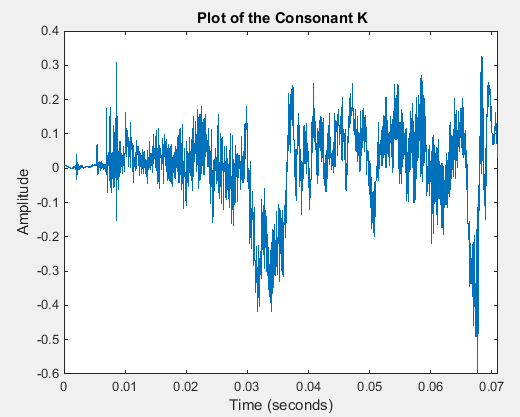Figure 5. Time vs. Amplitude Plot of Consonant K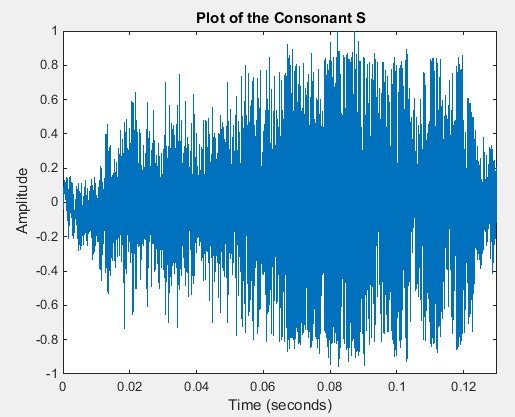Figure 6. Time vs. Amplitude Plot of Consonant S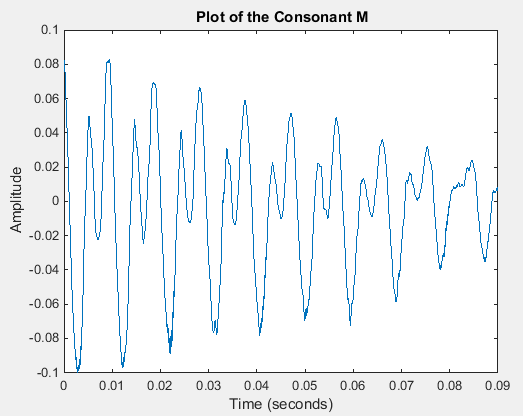Figure 7. Time vs. Amplitude Plot of Consonant M

Extracting and Plotting Syllabic and Consonantal Frequencies

Let us do the FFT of the entire word and do its power plot.

m_word_01 = length(word_01); %% window length
n_word_01 = pow2(nextpow2(m_word_01)); %% transform length
y_word_01 = fft(word_01, n_word_01); %% DFT of word_01
f_word_01 = (0:n_word_01-1)*(fs/n_word_01)/frf; %% frequency range
p_word_01 = y_word_01.*conj(y_word_01)/n_word_01; %% power
figure(8);
plot(f_word_01(1:floor(n_word_01/2)), p_word_01(1:floor(n_word_01/2)));
xlim([0 f_word_01(end)]);
xlabel('Frequency (Hz)');
ylabel('Power');
title('{\bf Component Frequencies of CALCIUM}');

Figure 8 gives the plot of the above FFT power analysis of the entire word.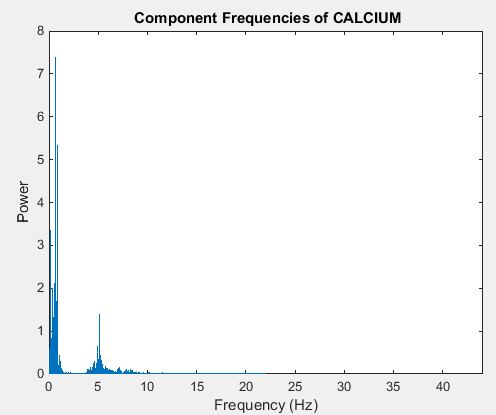Figure 8. Component Frequencies of the Word CALCIUM
There appear to be two spikes around 1 and 5. Let us investigate which spike comes from which syllable. The three MATLAB code segments below generate the plots shown in Figures 9, 10, and 11.

%% get the component frequencies of the syllable CAL
%% the left boundary is equal to time * fs
m_syl_01_word_01 = length(syl_01_word_01); %% window length
n_syl_01_word_01 = pow2(nextpow2(m_syl_01_word_01)); %% transform length
y_syl_01_word_01 = fft(syl_01_word_01, n_syl_01_word_01); %% DFT of the 1st syllable (KAL)
f_syl_01_word_01 = (0:n_syl_01_word_01-1)*(fs/n_syl_01_word_01)/frf; %% frequency range
p_syl_01_word_01 = y_syl_01_word_01.*conj(y_syl_01_word_01)/n_syl_01_word_01; %% power
figure(9);
plot(f_syl_01_word_01(1:floor(n_syl_01_word_01/2)), p_syl_01_word_01(1:floor(n_syl_01_word_01/2)));
xlim([0 f_syl_01_word_01(end)]);
xlabel('Frequency (Hz)');
ylabel('Power');
title('{\bf Component Frequencies of CAL}');
%%soundsc(syl_01_word_01, fs);

%% get the component frequencies of the syllable SI (second syllable)
%% the left boundary is equal to time * fs
m_syl_02_word_01 = length(syl_02_word_01); %% window length
n_syl_02_word_01 = pow2(nextpow2(m_syl_02_word_01)); %% transform length
y_syl_02_word_01 = fft(syl_02_word_01, n_syl_02_word_01); %% DFT of the second syllable
f_syl_02_word_01 = (0:n_syl_02_word_01-1)*(fs/n_syl_02_word_01)/frf; %% frequency range
p_syl_02_word_01 = y_syl_02_word_01.*conj(y_syl_02_word_01)/n_syl_02_word_01; %% power
figure(10);
plot(f_syl_02_word_01(1:floor(n_syl_02_word_01/2)), p_syl_02_word_01(1:floor(n_syl_02_word_01/2)));
xlim([0 f_syl_02_word_01(end)]);
xlabel('Frequency (Hz)');
ylabel('Power');
title('{\bf Component Frequencies of SI}');
%soundsc(syl_02_word_01, fs);

%% get the component frequencies of the syllable SI
%% the left boundary is equal to time * fs
m_syl_03_word_01 = length(syl_03_word_01); %% window length
n_syl_03_word_01 = pow2(nextpow2(m_syl_03_word_01)); %% transform length
y_syl_03_word_01 = fft(syl_03_word_01, n_syl_03_word_01); %% DFT of the 3rd syllable
f_syl_03_word_01 = (0:n_syl_03_word_01-1)*(fs/n_syl_03_word_01)/frf; %% frequency range
p_syl_03_word_01 = y_syl_03_word_01.*conj(y_syl_03_word_01)/n_syl_03_word_01; %% power
figure(11);
plot(f_syl_03_word_01(1:floor(n_syl_03_word_01/2)), p_syl_03_word_01(1:floor(n_syl_03_word_01/2)));
xlim([0 f_syl_03_word_01(end)]);
xlabel('Frequency (Hz)');
ylabel('Power');
title('{\bf Component Frequencies of UM}');
%soundsc(syl_03_word_01, fs);

Figures 9, 10, and 11 show the power plots of the syllables CAL, SI, and UM, respectively. The CAL plot shows a spike at frequency 1 and the SI plot shows the spike at frequency 5. The UM plot shows an insignificant spike at frequency 1.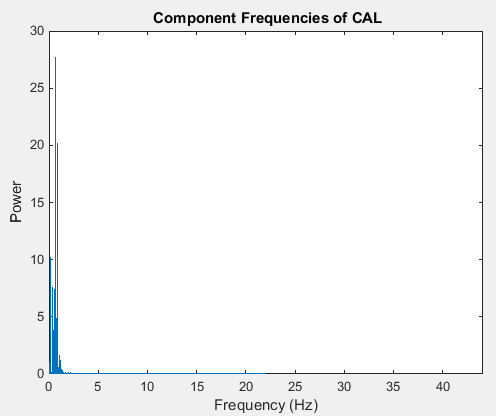Figure 9. Component Frequencies of CAL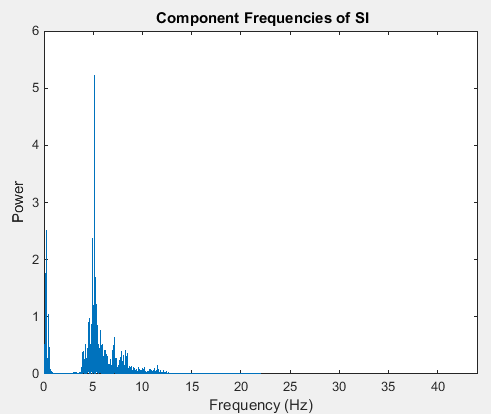Figure 10. Component Frequencies of SI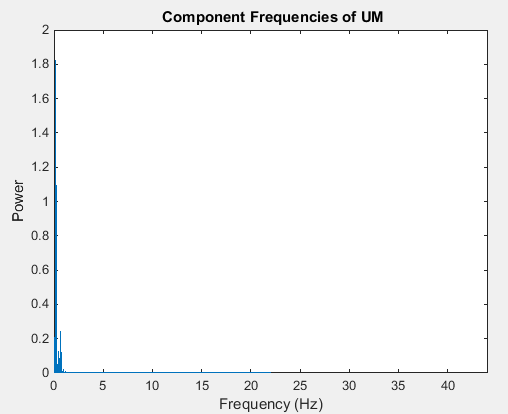Figure 11. Component Frequencies of UM

Extracting and Plotting the Consonants in Syllables CAL and SI

Since there are no significant spikes in the syllable UM, we can ignore it for the sake of simplicity and focus on the syllables CAL and SI. How much do the consonants K and S contribute to the frequency spikes shown in Figures 9 and 10?

%% get the component frequencies of the syllable K
%% the left boundary is equal to time * fs
m_k_word_01 = length(k_word_01); %% window length
n_k_word_01 = pow2(nextpow2(m_k_word_01)); %% transform length
y_k_word_01 = fft(k_word_01, n_k_word_01); %% DFT
f_k_word_01 = (0:n_k_word_01-1)*(fs/n_k_word_01)/frf; %% frequency range
p_k_word_01 = y_k_word_01.*conj(y_k_word_01)/n_k_word_01; %% power
figure(12);
plot(f_k_word_01(1:floor(n_k_word_01/2)), p_k_word_01(1:floor(n_k_word_01/2)));
xlim([0 f_k_word_01(end)]);
xlabel('Frequency (Hz)');
ylabel('Power');
title('{\bf Component Frequencies of K}');
%soundsc(k_word_01, fs);

%% get the component frequencies of the consonant S
%% the left boundary is equal to time * fs
m_s_word_01 = length(s_word_01); %% window length
n_s_word_01 = pow2(nextpow2(m_s_word_01)); %% transform length
y_s_word_01 = fft(s_word_01, n_s_word_01); %% DFT
f_s_word_01 = (0:n_s_word_01-1)*(fs/n_s_word_01)/frf; %% frequency range
p_s_word_01 = y_s_word_01.*conj(y_s_word_01)/n_s_word_01; %% power
figure(13);
plot(f_s_word_01(1:floor(n_s_word_01/2)), p_s_word_01(1:floor(n_s_word_01/2)));
xlim([0 f_s_word_01(end)]);
xlabel('Frequency (Hz)');
ylabel('Power');
title('{\bf Component Frequencies of S}');
%%soundsc(s_word_01, fs);
%soundsc(syl_02_word_01, fs);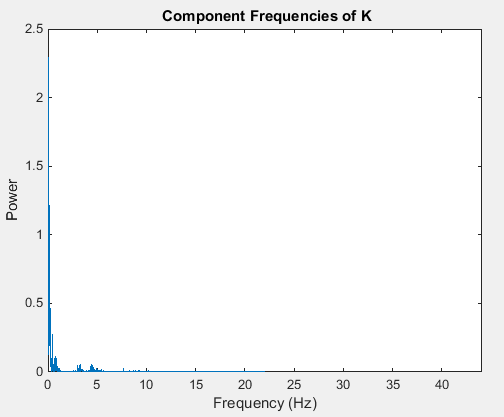Figure 12. Component Frequencies of K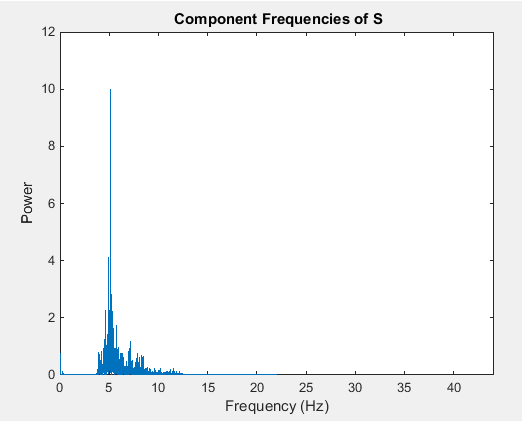Figure 13. Component Frequencies of S
Figure 12 seems to indicate that the frequency spike in CAL around frequency 1 is not caused by the consonant K but by the vowel A. Figure 13 seems to indicate that the frequency spike in the syllable SI around frequency 1 is caused by the consonant S.

MATLAB Source

This section gives the entire MATLAB source for easy of reference.
[x, fs] = audioread('calcium.wav'); %% x is the signal vector, fs is the frequency
x_01 = x(:,1); %% get the signal vector of the 1st channel
x_02 = x(:,2); %% get the signal vector of the 2nd channel

frf = 1000; %% frequency reduction factor

figure(1);
%% get the word 'calcium' on channel 01 and plot it
%% 5.51e4 and 8.89e4 are the rough estimates of the beginning and end
%% of the actual word. these boundaries are used to eliminiate silences
%% at the beginning and end of the file.
word_01 = x_01(5.51e4:8.89e4);
%% define the time line of the word. the time line goes from 0
%% upto (length(word_01)-1)/fs in increments of 1/fs. this is a pretty
%% common formula used in many audio processing applications.
t_word_01 = 0:1/fs:(length(word_01)-1)/fs;
%% plot the actual word against the defined time line.
plot(t_word_01, word_01);
xlabel('Time (seconds)');
ylabel('Amplitude');
title('{\bf Plot of the Word Calcium}')

%% get the first syllable and plot it
%% the left boundary is equal to time * fs
%% one can think of fs as speed. Then the whole audio vector
%% is a distance segment. To get a specific distance point in
%% the segment we multiply time by speed.
syl_01_word_01 = word_01(0.025*fs:0.26*fs);
t_syl_01_word_01 = (0:1/fs:(length(syl_01_word_01)-1)/fs);
figure(2);
plot(t_syl_01_word_01, syl_01_word_01);
xlim([0 t_syl_01_word_01(end)]);
xlabel('Time (seconds)');
ylabel('Amplitude');
title('{\bf Plot of the Syllable CAL}');
%soundsc(syl_01_word_01, fs);

%% get the second syllable & plot it
syl_02_word_01 = word_01(0.27*fs:0.51*fs);
t_syl_02_word_01 = (0:1/fs:(length(syl_02_word_01)-1)/fs);
figure(3);
plot(t_syl_02_word_01, syl_02_word_01);
xlim([0 t_syl_02_word_01(end)]);
xlabel('Time (seconds)');
ylabel('Amplitude');
title('{\bf Plot of the Syllable SI}');
%soundsc(syl_02_word_01, fs);

%% get the third syllable & plot it
syl_03_word_01 = word_01(0.51*fs:0.71*fs);
t_syl_03_word_01 = (0:1/fs:(length(syl_03_word_01)-1)/fs);
figure(4);
plot(t_syl_03_word_01, syl_03_word_01);
xlim([0 t_syl_03_word_01(end)]);
xlabel('Time (seconds)');
ylabel('Amplitude');
title('{\bf Plot of the Syllable UM}');
%soundsc(syl_03_word_01, fs);

%% get the consonant K in the syllable CAL (KAL) & plot it
k_word_01 = word_01(0.019*fs:0.09*fs);
t_k_word_01 = (0:1/fs:(length(k_word_01)-1)/fs);
figure(5);
plot(t_k_word_01, k_word_01);
xlim([0 t_k_word_01(end)]);
xlabel('Time (seconds)');
ylabel('Amplitude');
title('{\bf Plot of the Consonant K}');
%soundsc(k_word_01, fs);

%% get the consonant S in the syllable CI (SI) & plot it
s_word_01 = word_01(0.27*fs:0.40*fs);
t_s_word_01 = (0:1/fs:(length(s_word_01)-1)/fs);
figure(6);
plot(t_s_word_01, s_word_01);
xlim([0 t_s_word_01(end)]);
xlabel('Time (seconds)');
ylabel('Amplitude');
title('{\bf Plot of the Consonant S}');
%%soundsc(s_word_01, fs);

%% get the consonant M & plot it
m_word_01 = word_01(0.62*fs:0.71*fs);
t_m_word_01 = (0:1/fs:(length(m_word_01)-1)/fs);
figure(7);
plot(t_m_word_01, m_word_01);
xlim([0 t_m_word_01(end)]);
xlabel('Time (seconds)');
ylabel('Amplitude');
title('{\bf Plot of the Consonant M}');
soundsc(m_word_01, fs);

%% Get the component frequencies of the entire word
m_word_01 = length(word_01); %% window length
n_word_01 = pow2(nextpow2(m_word_01)); %% transform length
y_word_01 = fft(word_01, n_word_01); %% DFT of word_01
f_word_01 = (0:n_word_01-1)*(fs/n_word_01)/frf; %% frequency range
p_word_01 = y_word_01.*conj(y_word_01)/n_word_01; %% power
figure(8);
plot(f_word_01(1:floor(n_word_01/2)), p_word_01(1:floor(n_word_01/2)));
xlim([0 f_word_01(end)]);
xlabel('Frequency (Hz)');
ylabel('Power');
title('{\bf Component Frequencies of CALCIUM}');

%% get the component frequencies of the syllable CAL
%% the left boundary is equal to time * fs
m_syl_01_word_01 = length(syl_01_word_01); %% window length
n_syl_01_word_01 = pow2(nextpow2(m_syl_01_word_01)); %% transform length
y_syl_01_word_01 = fft(syl_01_word_01, n_syl_01_word_01); %% DFT of the 1st syllable (KAL)
f_syl_01_word_01 = (0:n_syl_01_word_01-1)*(fs/n_syl_01_word_01)/frf; %% frequency range
p_syl_01_word_01 = y_syl_01_word_01.*conj(y_syl_01_word_01)/n_syl_01_word_01; %% power
figure(9);
plot(f_syl_01_word_01(1:floor(n_syl_01_word_01/2)), p_syl_01_word_01(1:floor(n_syl_01_word_01/2)));
xlim([0 f_syl_01_word_01(end)]);
xlabel('Frequency (Hz)');
ylabel('Power');
title('{\bf Component Frequencies of CAL}');
%%soundsc(syl_01_word_01, fs);

%% get the component frequencies of the syllable SI (second syllable)
%% the left boundary is equal to time * fs
m_syl_02_word_01 = length(syl_02_word_01); %% window length
n_syl_02_word_01 = pow2(nextpow2(m_syl_02_word_01)); %% transform length
y_syl_02_word_01 = fft(syl_02_word_01, n_syl_02_word_01); %% DFT of the second syllable
f_syl_02_word_01 = (0:n_syl_02_word_01-1)*(fs/n_syl_02_word_01)/frf; %% frequency range
p_syl_02_word_01 = y_syl_02_word_01.*conj(y_syl_02_word_01)/n_syl_02_word_01; %% power
figure(10);
plot(f_syl_02_word_01(1:floor(n_syl_02_word_01/2)), p_syl_02_word_01(1:floor(n_syl_02_word_01/2)));
xlim([0 f_syl_02_word_01(end)]);
xlabel('Frequency (Hz)');
ylabel('Power');
title('{\bf Component Frequencies of SI}');
%soundsc(syl_02_word_01, fs);

%% get the component frequencies of the syllable SI
%% the left boundary is equal to time * fs
m_syl_03_word_01 = length(syl_03_word_01); %% window length
n_syl_03_word_01 = pow2(nextpow2(m_syl_03_word_01)); %% transform length
y_syl_03_word_01 = fft(syl_03_word_01, n_syl_03_word_01); %% DFT of the 3rd syllable
f_syl_03_word_01 = (0:n_syl_03_word_01-1)*(fs/n_syl_03_word_01)/frf; %% frequency range
p_syl_03_word_01 = y_syl_03_word_01.*conj(y_syl_03_word_01)/n_syl_03_word_01; %% power
figure(11);
plot(f_syl_03_word_01(1:floor(n_syl_03_word_01/2)), p_syl_03_word_01(1:floor(n_syl_03_word_01/2)));
xlim([0 f_syl_03_word_01(end)]);
xlabel('Frequency (Hz)');
ylabel('Power');
title('{\bf Component Frequencies of UM}');
%soundsc(syl_03_word_01, fs);

%% get the component frequencies of the syllable K
%% the left boundary is equal to time * fs
m_k_word_01 = length(k_word_01); %% window length
n_k_word_01 = pow2(nextpow2(m_k_word_01)); %% transform length
y_k_word_01 = fft(k_word_01, n_k_word_01); %% DFT
f_k_word_01 = (0:n_k_word_01-1)*(fs/n_k_word_01)/frf; %% frequency range
p_k_word_01 = y_k_word_01.*conj(y_k_word_01)/n_k_word_01; %% power
figure(12);
plot(f_k_word_01(1:floor(n_k_word_01/2)), p_k_word_01(1:floor(n_k_word_01/2)));
xlim([0 f_k_word_01(end)]);
xlabel('Frequency (Hz)');
ylabel('Power');
title('{\bf Component Frequencies of K}');
%soundsc(k_word_01, fs);

%% get the component frequencies of the consonant S
%% the left boundary is equal to time * fs
m_s_word_01 = length(s_word_01); %% window length
n_s_word_01 = pow2(nextpow2(m_s_word_01)); %% transform length
y_s_word_01 = fft(s_word_01, n_s_word_01); %% DFT
f_s_word_01 = (0:n_s_word_01-1)*(fs/n_s_word_01)/frf; %% frequency range
p_s_word_01 = y_s_word_01.*conj(y_s_word_01)/n_s_word_01; %% power
figure(13);
plot(f_s_word_01(1:floor(n_s_word_01/2)), p_s_word_01(1:floor(n_s_word_01/2)));
xlim([0 f_s_word_01(end)]);
xlabel('Frequency (Hz)');
ylabel('Power');
title('{\bf Component Frequencies of S}');
%%soundsc(s_word_01, fs);
%soundsc(syl_02_word_01, fs);

%% get the component frequencies of the consonant M
m_m_word_01 = length(m_word_01); %% window length
n_m_word_01 = pow2(nextpow2(m_m_word_01)); %% transform length
y_m_word_01 = fft(m_word_01, n_m_word_01); %% DFT
f_m_word_01 = (0:n_m_word_01-1)*(fs/n_m_word_01)/frf; %% frequency range
p_m_word_01 = y_m_word_01.*conj(y_m_word_01)/n_m_word_01; %% power
figure(14);
plot(f_m_word_01(1:floor(n_m_word_01/2)), p_m_word_01(1:floor(n_m_word_01/2)));
%%xlim([0 f_m_word_01(end)]);
xlabel('Frequency (Hz)');
ylabel('Power');
title('{\bf Component Frequencies of M}');
%soundsc(m_word_01, fs);
%soundsc(syl_02_word_01, fs);